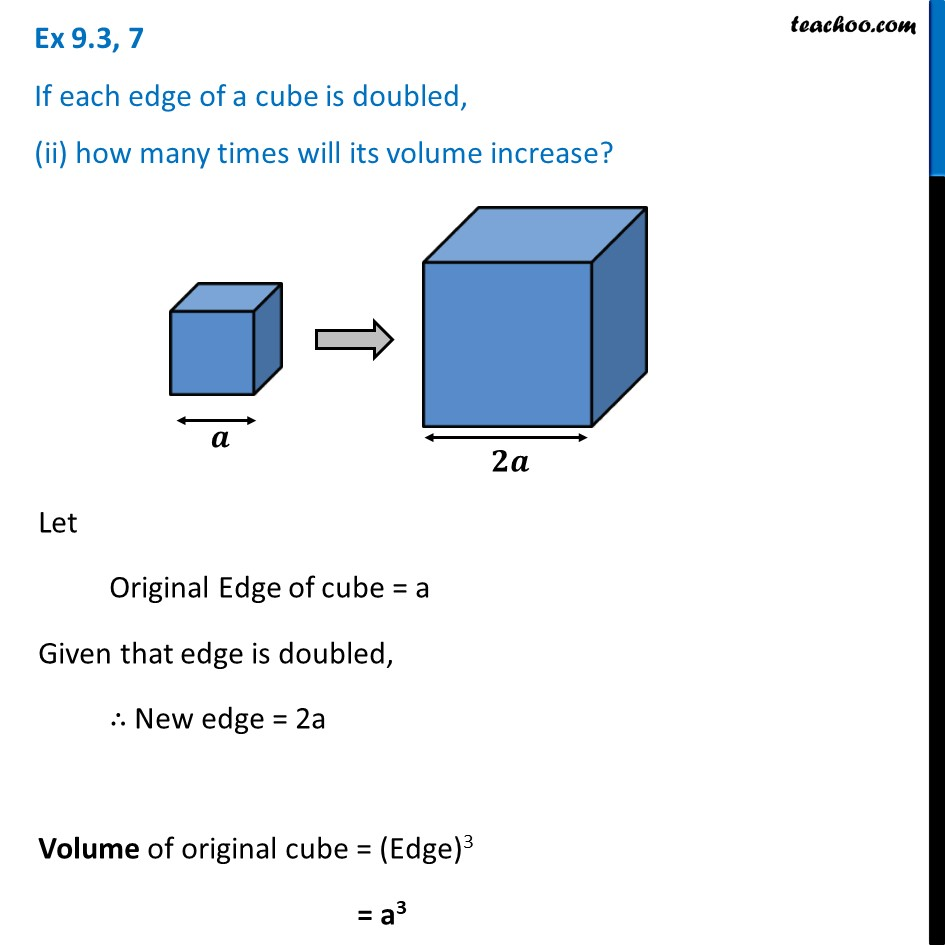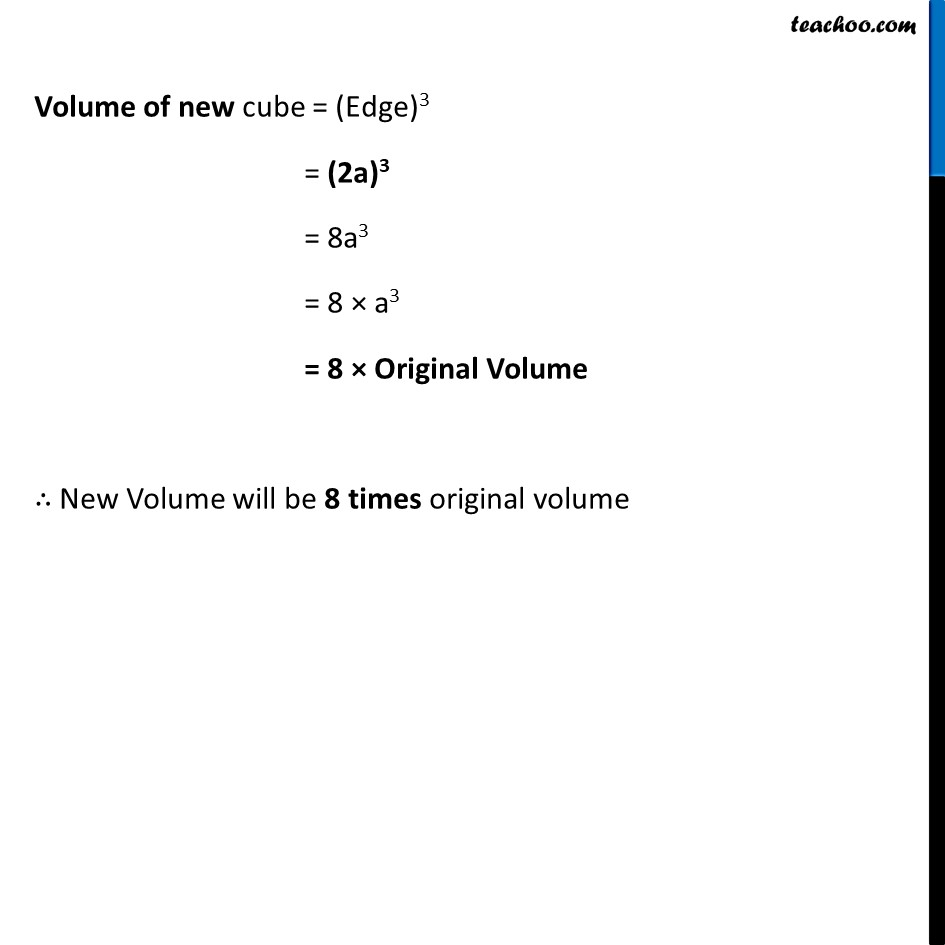Ex 9.3

Chapter 9 Class 8 Mensuration
Serial order wiseLearn in your speed, with individual attention - Teachoo Maths 1-on-1 Class

### Transcript

Ex 9.3, 7 If each edge of a cube is doubled, (ii) how many times will its volume increase? Let Original Edge of cube = a Given that edge is doubled, ∴ New edge = 2a Volume of original cube = (Edge)3 = a3 Surface area of new cube = 6 × (Edge)2 = 6 × (2a)2 = 6 × 4a2 = 4 × 6a2 = 4 × Original Surface Area ∴ New surface area will be 4 times original surface area.